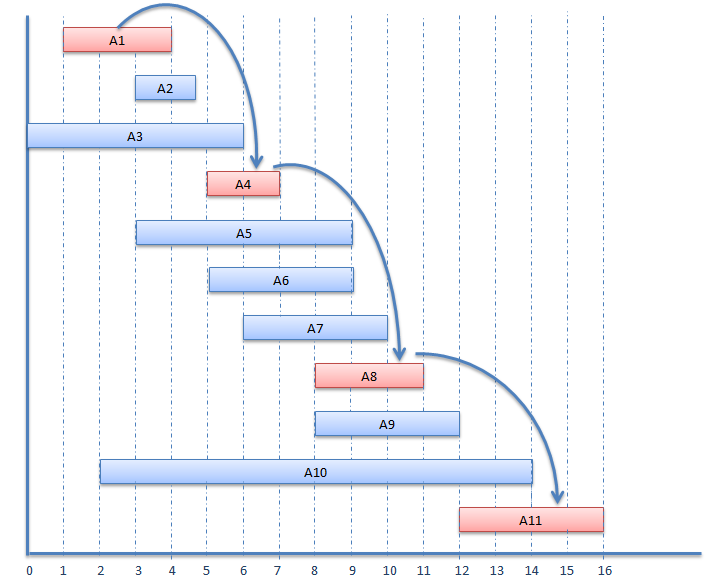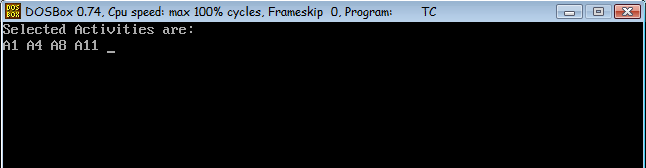Data Structure

# An activity selection problem

The activity selection problem is a mathematical optimization problem. That concerning the selection of non-conflicting activities. Each activity assigned by a start time (si) and finish time (fi). The activity selection problem is to select the maximum number of activities that can be performed by a single machine, assuming that a machine can only work on a single activity at a time.

### GREEDY ACTIVITY SELECTOR Algorithm

```GREEDY-ACTIVITY-SELECTOR(s, f)

1.  n ← length[s]
2.  A ← {a1}
3.  i ← 1
4.  for m ← 2 to n
5.  	do if sm ≥ fi
6.  	then A ← A U {am}
7.  	i ← m
8.  return A
```

### Example

Start time (si) finish time (fi) Activity name
1 4 A1
3 5 A2
0 6 A3
5 7 A4
3 9 A5
5 9 A6
6 10 A7
8 11 A8
8 12 A9
2 14 A10
12 16 A11Selected Activities are: A1, A4, A8, A11

### activity selection problem in c

```
#include<stdio.h>

#include<conio.h>

void activities(int s[], int f[], int n)

{

int i, j;

printf ("Selected Activities are:\n");

i = 1;

printf("A%d ", i);

for (j = 1; j < n; j++)

{

if (s[j] >= f[i])

{

printf ("A%d ", j+1);

i = j;

}

}

}

void main()

{

int s[] =  {1, 3, 0, 5, 3, 5, 6, 8, 8, 2, 12};

int f[] =  {4, 5, 6, 7, 9, 9, 10, 11, 12, 14, 16};

int n = sizeof(s)/sizeof(s);

clrscr();

activities(s, f, n);

getchar();

getch();

}

```

#### Output